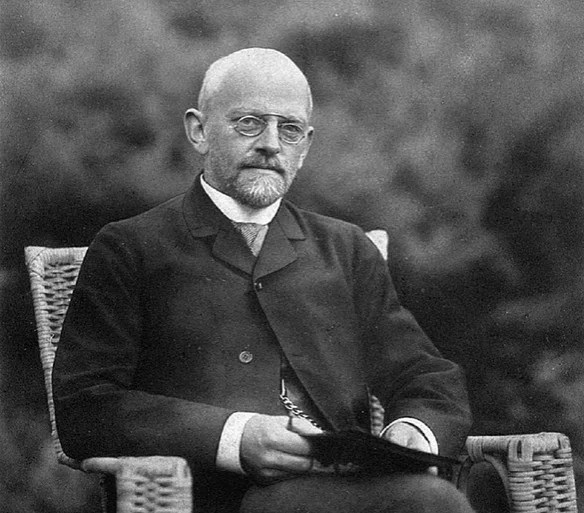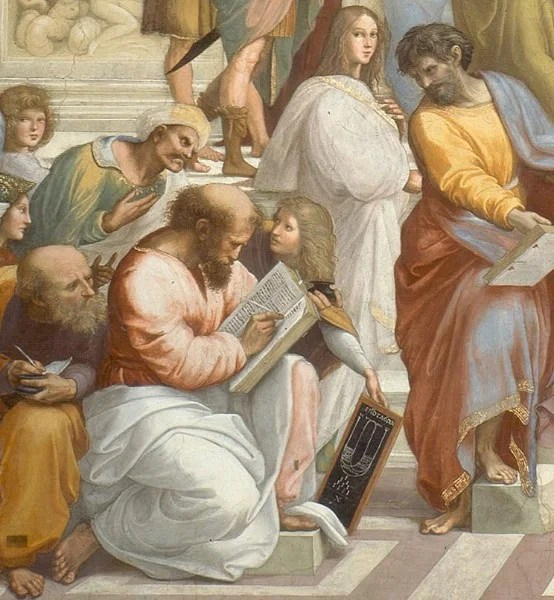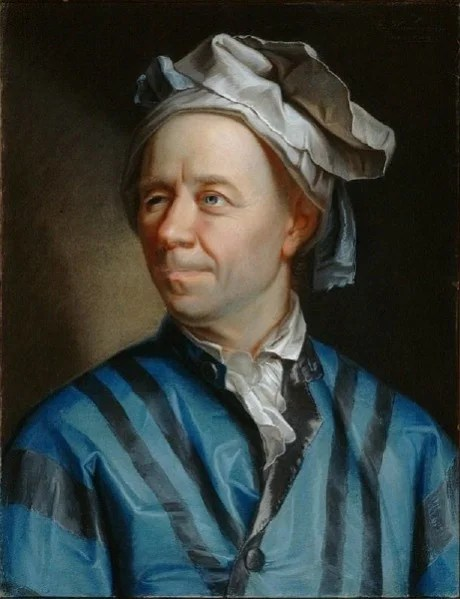# 16 Famous and Greatest Mathematicians | 2020 Edition

Who are the greatest and most famous mathematicians ever lived? Well, its answer is not easy as mathematics was known to humanity since the prehistoric times, long before the birth of Christ.

The role of mathematics in our lives is immense. Math made it possible to transmit the electricity over thousands of kilometers, helped explore the concept of DNA, gave rise to computers, and it is essential in our quest to better understand the universe.

Without math, scientists can’t develop better medicines and engineers can’t explore new technologies. The list goes on and on.

Like most things, the mathematics we know today didn’t just randomly came into existence. It takes decades for mathematicians to formulate a new, groundbreaking theorem and equation. So who are these mathematicians? Let’s find out.

### 16. Srinivasa RamanujanKnown For: Ramanujan–Petersson conjecture; Ramanujan’s master theorem

Srinivasa Ramanujan was perhaps the most remarkable mathematician in modern India. Although Ramanujan had no formal training, his advanced mathematical knowledge at a very young age left many completely awestruck.

By the age of 16, he was able to study Bernoulli numbers, which he had developed himself, and calculated the Euler–Mascheroni constant. Before his death at a young age of 32, Ramanujan had successfully assembled almost 4,000 different mathematical identities.

He gained international fame after G. H Hardy, a prominent British mathematician, recognized his work and compared him with the likes of Euler and Jacobi.

### 15. Joseph-Louis LagrangeKnown For: Lagrangian mechanics; Celestial Mechanics; Number Theory

Joseph Lagrange was one of the most notable students of the great Leonhard Euler. Lagrange started his mathematical career with variational calculus (in 1754), which led to the formulation of Euler–Lagrange equation.

Lagrange reformulated classical mechanics to introduce Lagrangian Mechanics a few years later. His famous work on analytical mechanics (Mécanique analytique) helped other researchers develop the field of mathematical physics.

### 14. Andrew WilesAccolades: Wolf Prize (1995/6); Abel Prize (2016)

Sir Andrew John Wiles is a British mathematician most famous for proving Fermat’s Last Theorem, once considered the “most difficult mathematical problem”.

In 1975, under the guidance of John H. Coates, Andrew Wiles started working on Iwasawa theory, which he continued with American mathematician Barry Mazur.

His biggest breakthrough, however, came in the early 1990s, when he was able to prove most of the modularity theorem (formerly the Taniyama–Shimura conjecture). The modularity theorem, in essence, is related to Fermat’s Last Theorem and was enough to prove it.

Mr. Wiles is currently serving as a research professor at the University of Oxford.

### 13. Carl Gustav Jacob JacobiKnown For: Jacobi’s elliptic functions; Jacobi transform

Carl Gustav Jacobi was one of the prominent mathematicians of the 19th century. His formulation of the theory of elliptic functions is perhaps his greatest contribution to the field. Jacobi was also instrumental in the studies of differential equations and rational mechanics (Hamilton-Jacobi theory).

Moreover, he made fundamental contributions in the fields of mechanical dynamics and number theory.

### 12. Alan TuringKnown For: Cryptanalysis of the Enigma; Turing’s proof

During the Second World War, the German intelligence network was considered almost impenetrable. Many allied nations feared that if they cannot intercept important transmissions by the Nazi high command, they might eventually lose the war.

It was Alan Turing, who with his unprecedented mathematical and cryptanalytic abilities made significant improvements over the Polish-made bombe and devised a machine that can decode the Enigma faster.

After the end of the war, Turing joined the National Physical Laboratory (U.K), where he designed the Automatic Computing Engine, one of the earliest stored-program computer.

Later in his career, he diverted his attention to theoretical biology. It was during this time that he predicted (mathematically) the Belousov–Zhabotinsky reaction, which was later observed in the 1960s.

### 11. G.F. Bernhard RiemannBernhard Riemann

Known For: Riemann integral; Fourier series

Georg Bernhard Riemann was born in a small village near Dannenberg, Germany. Under the tutelage of Carl Friedrich Gauss, Riemann studied differential geometry and came up with his theory of additional or higher dimensions. His work is now known as Riemannian geometry.

Riemann was heavily influenced by Johann Gustav Dirichlet, who also made an impact on his mathematical career. Only by using the Dirichlet principle, he was able to formulate the famous Riemann mapping theorem.

Some of his mathematical equations were later used by Einstein in his General Relativity theory.

### 10. Henri PoincaréHenry Poincaré along with Marie Curie at the 1911 Solvay Conference

Known For: Three-body problem; Chaos theory; Poincaré–Hopf theorem

According to Eric Bell, a notable Scottish Mathematician, Henri Poincare was probably one of the last Universalist, as he thrived in almost all known fields of mathematics at that time.

Poincare during his lifetime contributed numerous theories in fields of mathematical physics, applied mathematics, and astronomy. He was instrumental in the formulation of the theory of Special Relativity.

Moreover, his exceptional works on Lorentz transformation and the Three-body problem paved way for mathematicians as well as astrophysicists to make discoveries about our planet and the outer space. His theoretical works even inspired famous artists such as Picasso and Braque to establish an art movement (Cubism) in the 20th century.

### 9. David HilbertDavid Hilbert in his Göttingen home | Image Courtesy: idw-online.de

Known For: Proof theory; Hilbert’s problems

David Hilbert was perhaps the most famous mathematician of time. He was instrumental in developing fundamental theories in the field of commutative algebra, calculus of variations, and mathematical physics.

Hilbert’s problems (a set of twenty-three mathematical problems, which he published in 1900) influenced groundbreaking studies in different fields of mathematics. Some of those problems remain unsolved to this date.

In his later days, David Hilbert devoted himself to physics. It was during this time he competed against Albert Einstein on general relativity.

### 8. FibonacciKnown For: Fibonacci numbers

Fibonacci, also known as Leonardo of Pisa, was one of the most accomplished mathematicians of the high middle ages.

Perhaps his most important contribution to the subject is Liber Abaci, a personal book, through which he popularized the Indo-Arabic numeral system (0,1,2,3,4..) and Fibonacci sequence in Europe.

Fibonacci sequence is used in computer algorithms and databases.

### 7. Bernoulli FamilyIn the world of mathematics, the Bernoulli family holds a very special place. Originally from Antwerp (Belgium), Jacob and his brother Johann Bernoulli were the first mathematicians in this family.

Both Jacob and Johann worked together on infinitesimal calculus and are credited for theorems and justifications such as Bernoulli numbers and Brachistochrone curve.

Daniel Bernoulli, Jacob’s son, was one of the most prominent members of the Bernoulli family. His most acclaimed work, the Bernoulli’s principle, mathematically explains the workings of a carburetor and an airplane wing. He also made substantial contributions in the fields of probability and statistics.

### 6. PythagorasPythagoras (writing a book) depicted by Raphael’s fresco The School of Athens

Known For: Pythagorean theorem; Theory of Proportions

Pythagoras of Samos was born in around 570 BC. Like most ancient Greeks, not much is known about his early life. As a philosopher, his works influenced the likes of Plato and Aristotle as well as Johannes Kepler and Isaac Newton.

Although its authenticity remains debatable, many mathematical findings are attributed to Pythagoras. Perhaps the most famous of them is the Pythagoras theorem (named after him). Many historians have stated the theorem was known by the Babylonians well before the time of Pythagoras.

Pythagoras may have also been responsible for the discovering Theory of Proportions.

### 5. Carl Friedrich GaussAccolades: Lalande Prize (1809), Copley Medal (1838)

Carl Friedrich Gauss was perhaps the most influential mathematician since the Ancient Greeks. His contributions in various fields of mathematics and physics are almost second to none. Gauss started showing signs of brilliance at an early age of seven when he could solve arithmetic progressions much faster than anyone in his class.

Some of his popular works include Gauss’ Law and Theorema Egregium, which concluded that the Earth cannot be displayed on a map without distortion. He was the first to speculate the possibility of non-Euclidean geometry, although his works were never published.

### 4. Issac NewtonKnown For: Newton’s Laws of Motion; Calculus; Newtonian Mechanics

Sir Issac Newton is one of the founding fathers of classical mechanics as well as infinitesimal calculus. His views on gravity remained universally accepted until Einstein’s theory of relativity.

Newton’s most remarkable contribution to mathematics is calculus (then called infinitesimals), which he developed independently of his contemporary Gottfried Wilhelm Leibniz.

It was Newton who first explained the reason behind tidal disturbances on Earth and helped validate Kepler’s laws of planetary motion. His works on optics gave us the first-ever refracting telescope.

### 3. Leonhard EulerA portrait of Leonhard Euler by Jakob Emanuel Handmann

Known For: Euler’s conjectures; Euler’s equations; Euler’s numbers

In a tribute to Leonhard Euler’s contributions toward mathematics, Pierre-Simon Laplace, a notable French astronomer and mathematician wrote, “Read Euler, read him again and again, he is the master of us all.”

Mathematicians today, holds Euler in the highest regard and consider him the most important mathematicians of the 18th century.

Euler made significant contributions to almost every major fields in mathematics including algebra, trigonometry, and geometry. In physics, his works on fluid dynamics and Fourier series is unmatched.

### 2. ArchimedesKnown For: Archimedes’ principle; Hydrostatics

Born in around 287 BC, in Syracuse, Sicily, Archimedes was well versed in mathematics, physics, and astronomy of that time. He was a polymath. However, most of his literary works have not survived.

Archimedes was one of the pioneers of geometry, who derived formulas for area of a circle, volume and surface area of a sphere. His method of determining the value of pi remained unchallenged and the only known way to calculate the circumference of a circle for decades.

The Fields Medal, the highest honor in the field of mathematics, carries a portrait of (right facing) Archimedes along with a quote attributed to him.

“Transire suum pectus mundoque potiri” — Rise above oneself and grasp the world.

### 1. EuclidKnown For: Euclidean geometry; Euclidean algorithm

Euclid of Alexandria was a Greek mathematician, who is widely regarded as the founder of geometry. Euclid’s Elements, a compilation of 13 books, is considered to be one of the oldest and most influential books on mathematics.

Although geometry (which is now known as the Euclidean geometry) is the focal point in Euclid’s Elements, it also features a comprehensive introduction of the elementary number theory. His works on optics have also been widely acknowledged.

Euclid’s systematic approach in his work — starting from axioms and then logically obtaining complex results, has influenced some of the greatest minds of later generations. Newton’s Principia Mathematica is a perfect example of it.Written by
###### Varun Kumar

Varun Kumar is a professional science and technology journalist and a big fan of AI, machines, and space exploration. He received a Master's degree in computer science from Indraprastha University. To find out about his latest projects, feel free to directly email him at [email protected]

•TWAHIRWA JOSUE says:

•RoyAlbrecht says:

Where have you been living…, ? under a rock perhaps?
Einstein was “…outted…” decades ago as a plagiaristic Jew and used by the Jew World Order to make Jews look smarter than everyone else…
Jews control the mass media and therefore use it to convince the masses that theya re the victims, when in fact they are the criminals.

•Arnab jana says:

World famous physicist & mathematican

•DEVASHISH says:

what about Arayabhata?????? he discovered the 0 as well as the decimals